• 在工程分析中，人们对结构的应力值感兴趣。 此代码显示了如何从位移中获得应力值。 高斯点处的应力值是准确的，这些应力用于获得节点处的应力。 可以使用外推或补丁恢复等方法。 对获得的应力求平均以获得平均应力。...matlab
• 本文介绍了图像全息法在光弹性应力分析中的应用。实验和理论研究的结果都说明由氦氖气体激光器发出的非偏振光是和圆偏振光等效的。用这个方法，可以通过补偿器测定等和线分数条纹，可以消除条纹图案的视差和浮动，...
• 对交通部公路桥涵标准图中的 23个单跨石拱桥的计算与分析表明:共旋坐标法四边形平面应力单元的列式、程序和求解器具有较强的非线性分析能力,能较好地分析石拱桥受力全过程的非线性性能。该计算结果提供了对中小跨度...
• https://www.zhihu.com/question/30439292 plate stress plate strain
https://www.zhihu.com/question/30439292
http://blog.sina.cn/dpool/blog/s/blog_c4c804690102vqqs.html
plate stress
plate strain


展开全文• 使用二阶三角形元素进行平面应力和应变条件的有限元分析matlab
• 解决了平面应力问题，即边缘均匀受力的板。使用等参Q4元素将板离散化。使用标准的FEM软件进行预处理。使用二阶高斯积分获得刚度矩阵。将获得的结果与标准FEM软件进行比较，两者的结果吻合良好。 代码获取...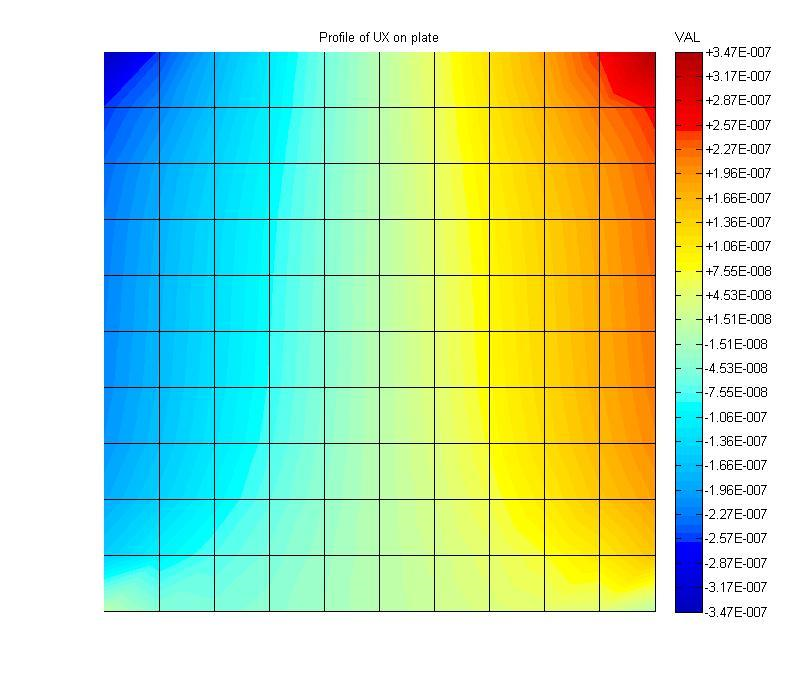使用有限元法求解边缘均匀受拉的板

解决了平面应力问题，即边缘均匀受力的板。使用等参Q4元素将板离散化。使用标准的FEM软件进行预处理。使用二阶高斯积分获得刚度矩阵。将获得的结果与标准FEM软件进行比较，两者的结果吻合良好。

代码获取 ​​​​​​​


展开全文• midas的工程概况-平面基坑应力渗流耦合分析，有详细的步骤。Midas
• 大型InSb焦平面阵列探测器在热冲击下的建模与应力分析研究论文
• 算例如上所述，这是典型的平面应力问题，用ANSYS Mechanical APDL软件对其开展分析，以ANSYS 17.0版本为例，具体过程如下： 0. 打开软件 开始 -> ANSYS 17.0 -> Mechanical APDL 17.0 1. 初始设置 1.1 ...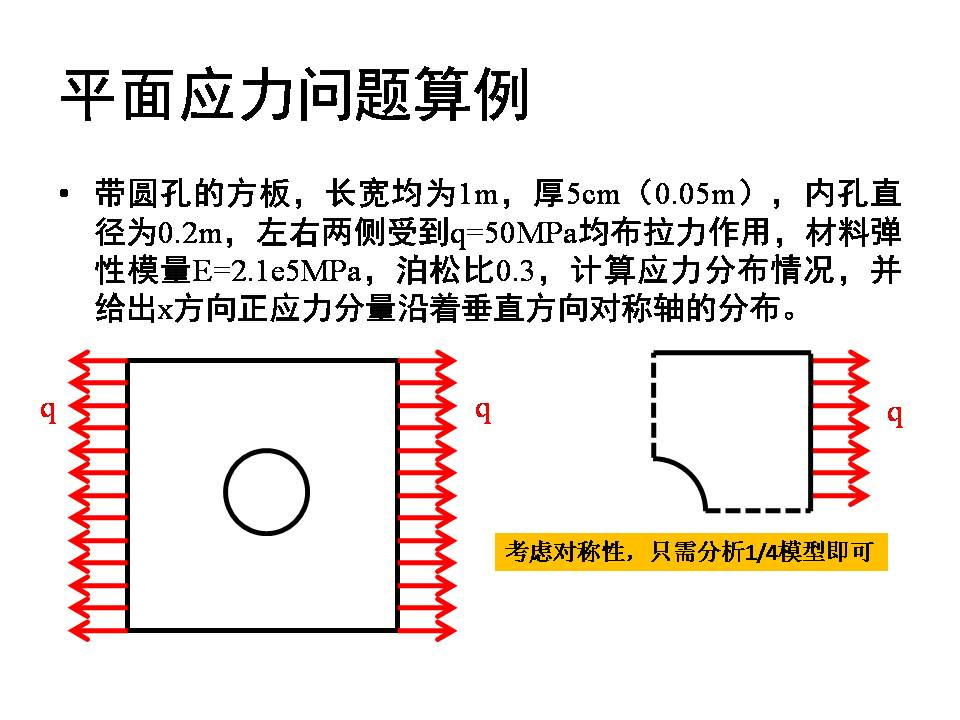算例如上所述，这是典型的平面应力问题，用ANSYS Mechanical APDL软件对其开展分析，以ANSYS 17.0版本为例，具体过程如下：
0. 打开软件
开始 -> ANSYS 17.0 -> Mechanical APDL 17.0
1. 初始设置
1.1 设置工作路径
Utility Menu中，File -> Change Directory …，在“浏览文件夹”对话框中选择想要存放该工作的文件夹，然后确定。
1.2 设置工作文件名
Utility Menu中，File -> Change Jobname …，在“Change Jobname”对话框中更改该工作的名字为“planeStress”，后OK确定。
1.3 设置工作标题
Utility Menu中，File -> Change Title …，在“Change Title”对话框中输入标题“Analysis of a square plate with a circular hole”，OK确定。
1.4 设定分析模块
2. 前处理
2.1 单位定义
ANSYS Mechanical APDL中无需指定单位，只需要保证物理量量纲统一即可。
2.2 定义单元类型
在Library of Element Types对话框中选择单元类型为Solid，Quad中的4 node 182，然后OK，关闭Library of Element Types对话框。
可见Element Types对话框中已经把该单元包含进来了，打开Options…选项，确定K3选项中是Plane stress，平面应力设置。OK关闭设置，Close关闭Element Types对话框。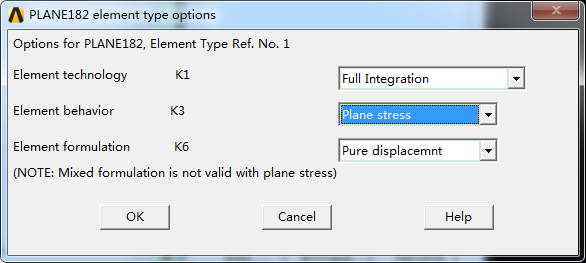2.3 定义材料属性
Main Menu中，Preprocessor -> Material Props -> Material Models，
在打开的Define Material Model Behavior窗口中，选择Structural -> Linera -> Elastic -> Isotropic，
在打开的对话框中的EX输入弹性模量为2.1e11，单位为Pa，PRXY为泊松比，指定为0.3，OK确认。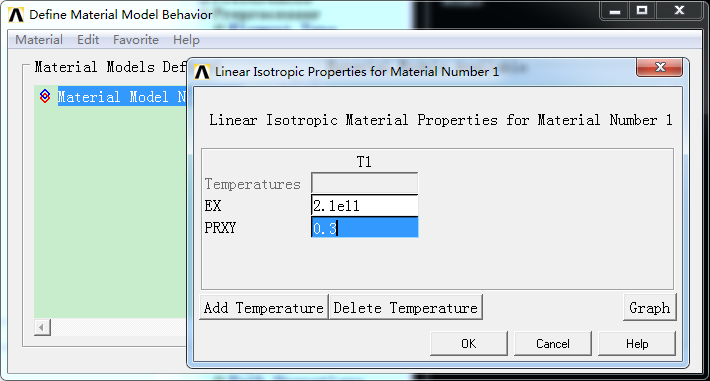2.4 创建模型
（a）创建关键点
Main Menu中，Preprocessor -> Modeling -> Create -> Keypoints -> In Active CS，
在Create Keypoints in Active Coordinate System对话框中，
输入节点编号1，坐标0，0，0，Apply；
输入节点编号2，坐标0.1，0，0，Apply；
输入节点编号3，坐标0，0.1，0，Apply；
输入节点编号4，坐标0.5，0，0，Apply；
输入节点编号5，坐标0，0.5，0，Apply；
输入节点编号6，坐标0.5，0.5，0，Apply；
输入节点编号7，坐标0.0707106781186548，0.0707106781186548，0，Apply；
输入节点编号8，坐标0.05，0.0866025403784439，0，Apply；
输入节点编号9，坐标0.0866025403784439，0.05，0，Apply；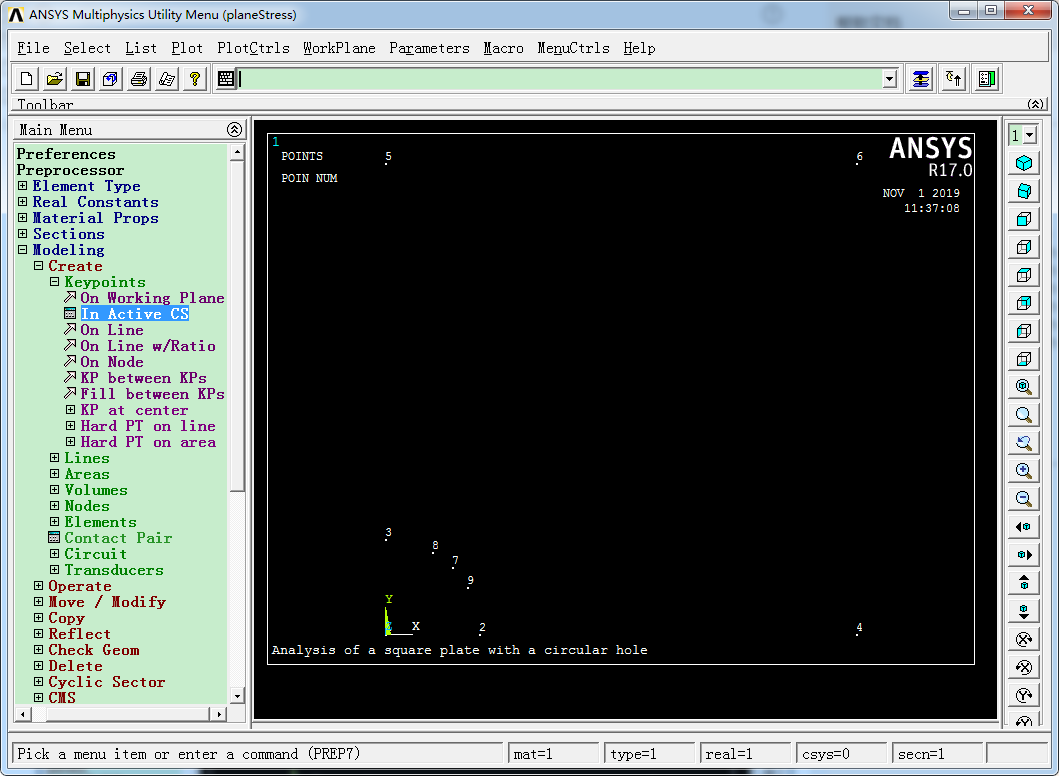（b）创建线
Main Menu中，Preprocessor -> Modeling -> Create -> Lines -> Lines -> Straight Line，
在Create Straight Line对话框中，或者直接输入2,4（注意是英文逗号），Apply，即可生成从关键点2到关键点4的线1了。
同样方法，生成3-5、4-6、5-6的直线。
Main Menu中，Preprocessor -> Modeling -> Create -> Lines -> Arcs -> Through 3 KPs，
输入2,7,9，即起点、终点、中间点。OK。
同样的方法，生成过7,3,8点的圆弧，OK。
哦，还得生成一条过7,6点的直线呢。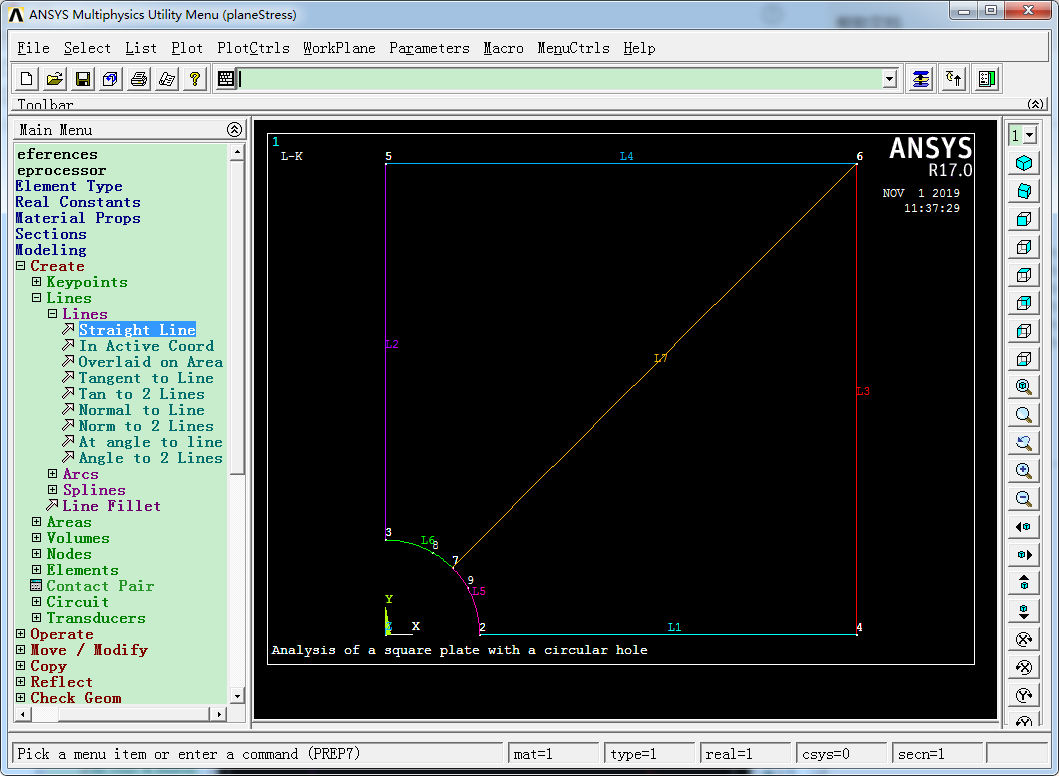（c）生成面
Main Menu中，Preprocessor -> Modeling -> Create -> Areas -> Arbitirary -> By Lines，
用L1、L3、L7、L5生成Area1；
用L6、L7、L4、L2生成Area2。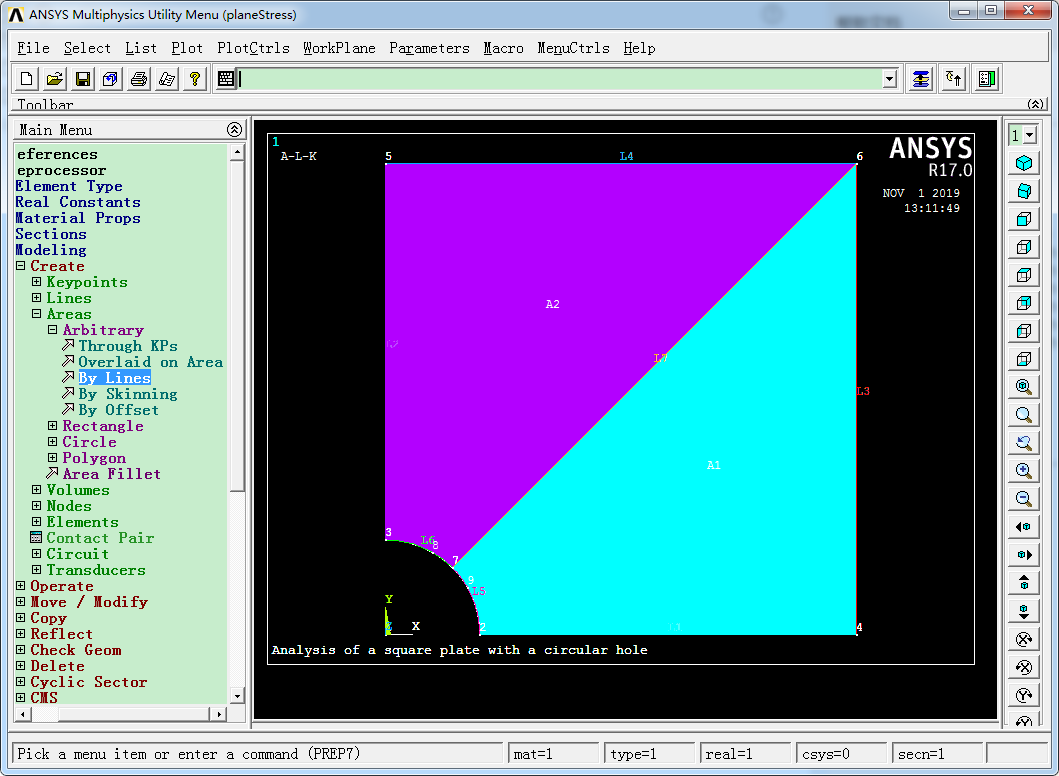至此，模型生成完毕。
2.5 划分单元
Main Menu中，Preprocessor -> Meshing -> MeshTool，
在弹出的MeshTool对话框中，先确定网格尺度，点击Size Controls组类中Lines后面的Set，
在弹出的Element Size on Picked Lines对话框中，选择L3、L4、L5、L6，OK，
在弹出的Element Sizes on Picked Lines对话框中，设定单元划分数目NDIV为20，OK确定。
同样方法，将L1、L2、L7的网格数目NDIV设置为40。
Main Menu中，Preprocessor -> Meshing -> MeshTool，
即可完成网格划分。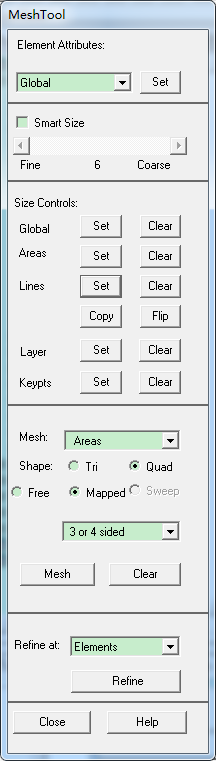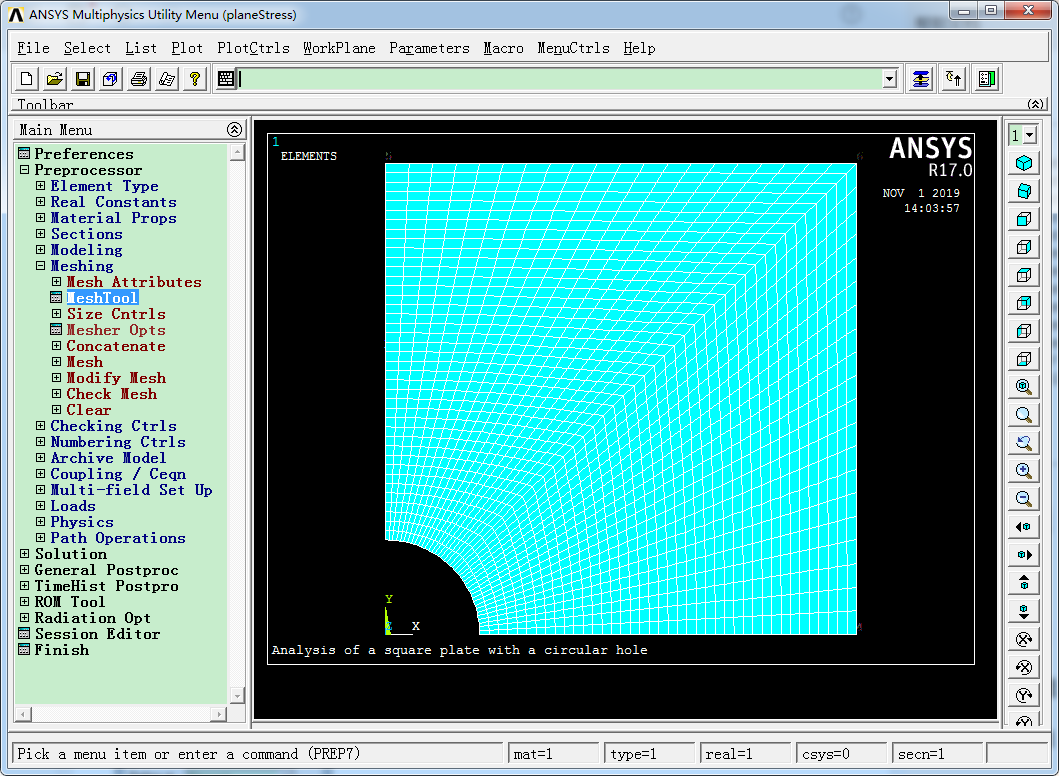3. 求解
3.1 设置分析类型
Main Menu中，Solution -> Analysis Type -> New Analysis，保持默认的Static静态分析，OK。
3.2 施加约束（施加边界条件）
Main Menu中，Solution -> Define Loads -> Apply -> Structural -> Displacement -> On Lines，
在弹出的Apply U,ROT on Lines选择框中，用鼠标在图形窗口中点选L1，即最下部的水平直线段，OK，
在弹出的Apply U,ROT on Lines对话框中，DOFs to be constrained（限定自由度）选项中，选中UY，即限制该线上节点在Y方向的位移，OK确定。
同样的方法，将L2，即最左侧竖直线的UX设置为0。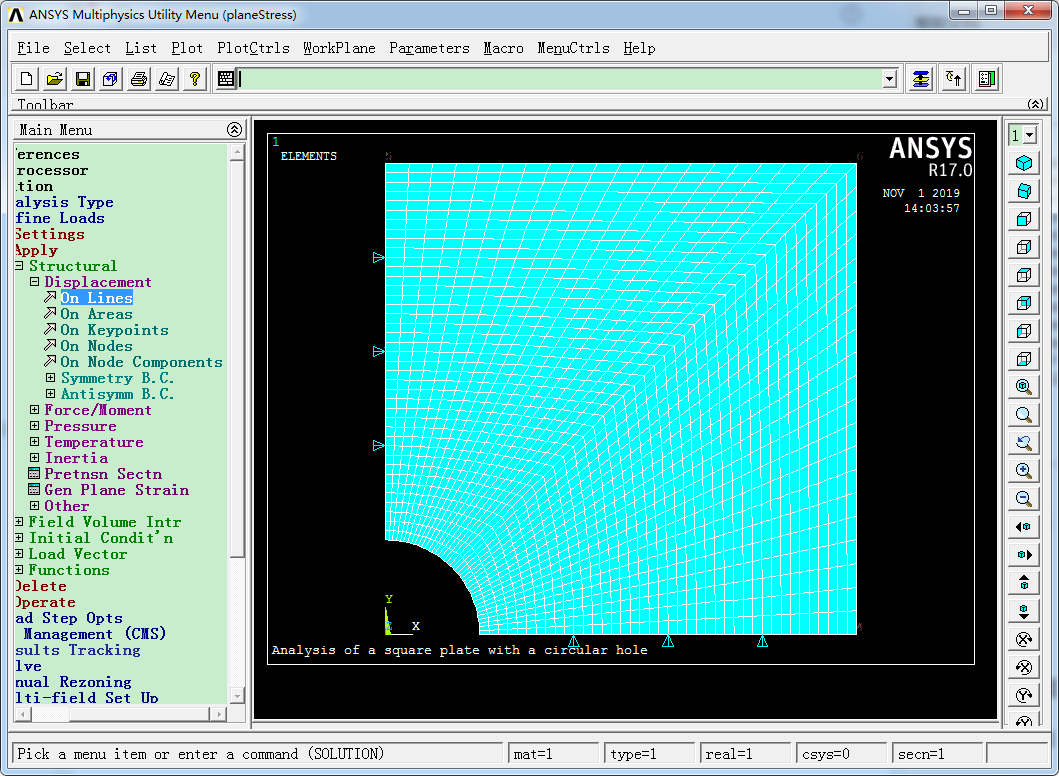3.3 施加载荷
Main Menu中，Solution -> Define Loads -> Apply -> Structural -> Pressure -> On Lines，
跳出的Apply Pres on Lines窗口中用鼠标拾取最右侧竖直线L3，OK，
跳出的Apply Pres on Lines窗口中选择FY，输入-50e6，单位为Pa，OK，注意这里有个负号，表示其方向为垂直于边界线朝外！
可见对应右侧竖直线上已经用红色箭头标出了添加好的均布载荷。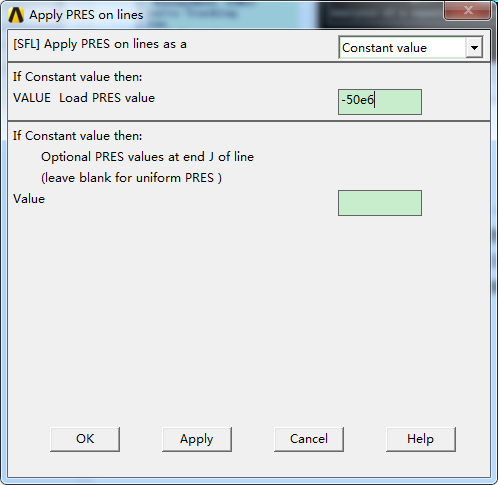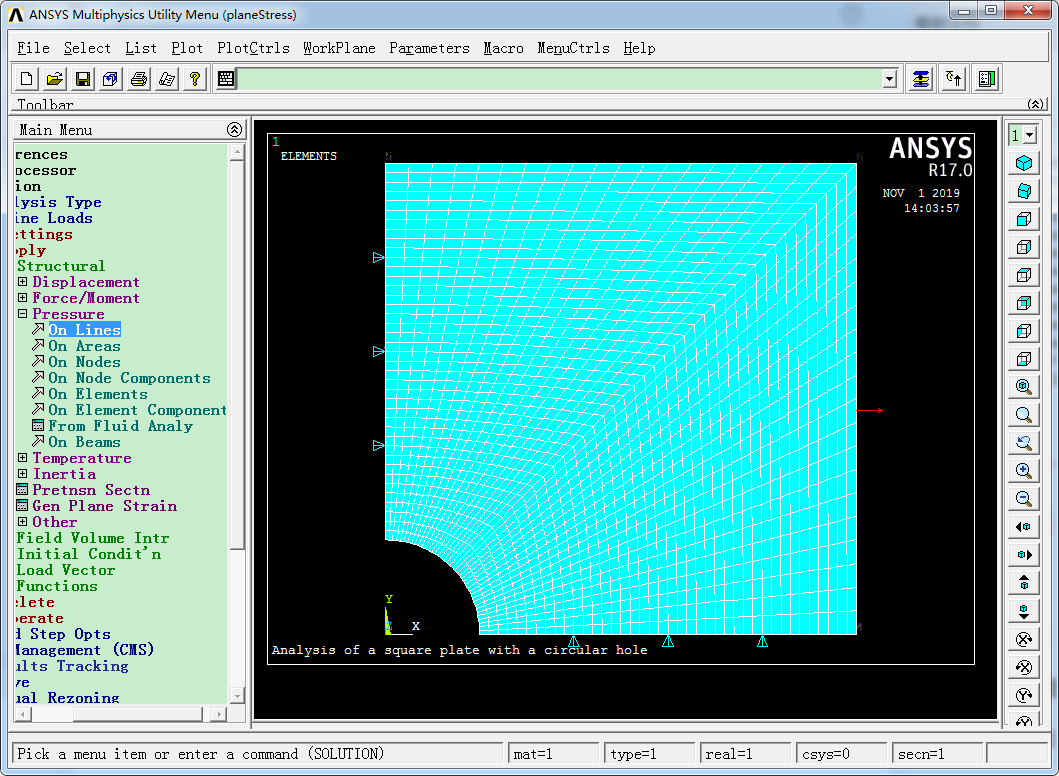3.4 求解
Main Menu中，Solution -> Solve -> Current LS，
注意：如果没有展开计算，提示错误，那可能是前面某个地方没有设置好，比如边界条件没有完全施加，外载荷没有施加，截面面积未给定，弹性模量没指定等。
4 后处理
4.1 查看变形状态
Main Menu中，General PostProc -> Plot Results -> Deformed Shape，
在Plot Deformed Shape窗口中，选择Def + undef edge，OK。
不难发现，在外部均布拉伸载荷作用下，板中心的圆孔变形成了椭圆孔，板外边界在x方向是拉伸，在y方向是压缩的。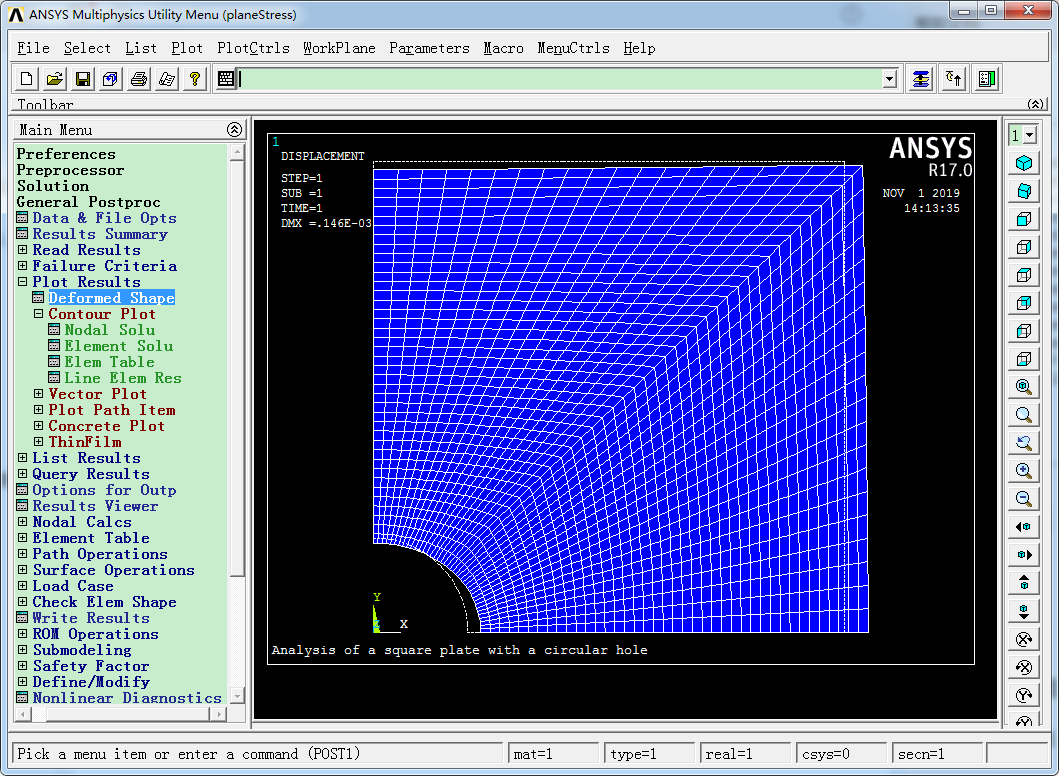4.2 绘制变形和应力云图
Main Menu中，General PostProc -> Plot Results -> Contour Plot -> Nodal Solu，
选择Stress中的X-Component of stress，OK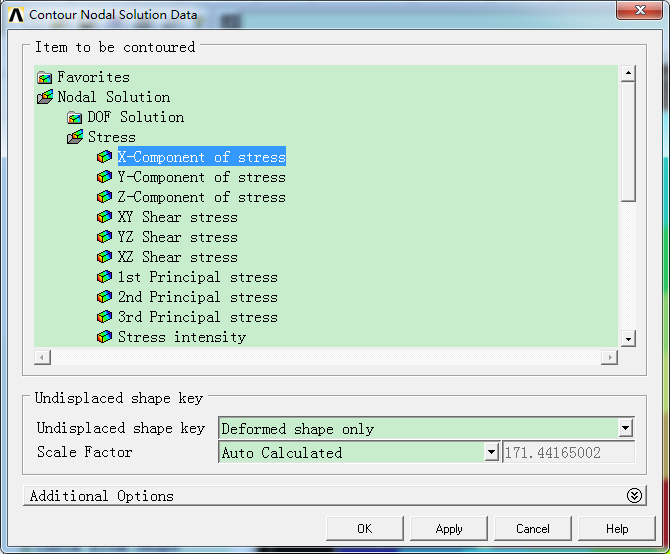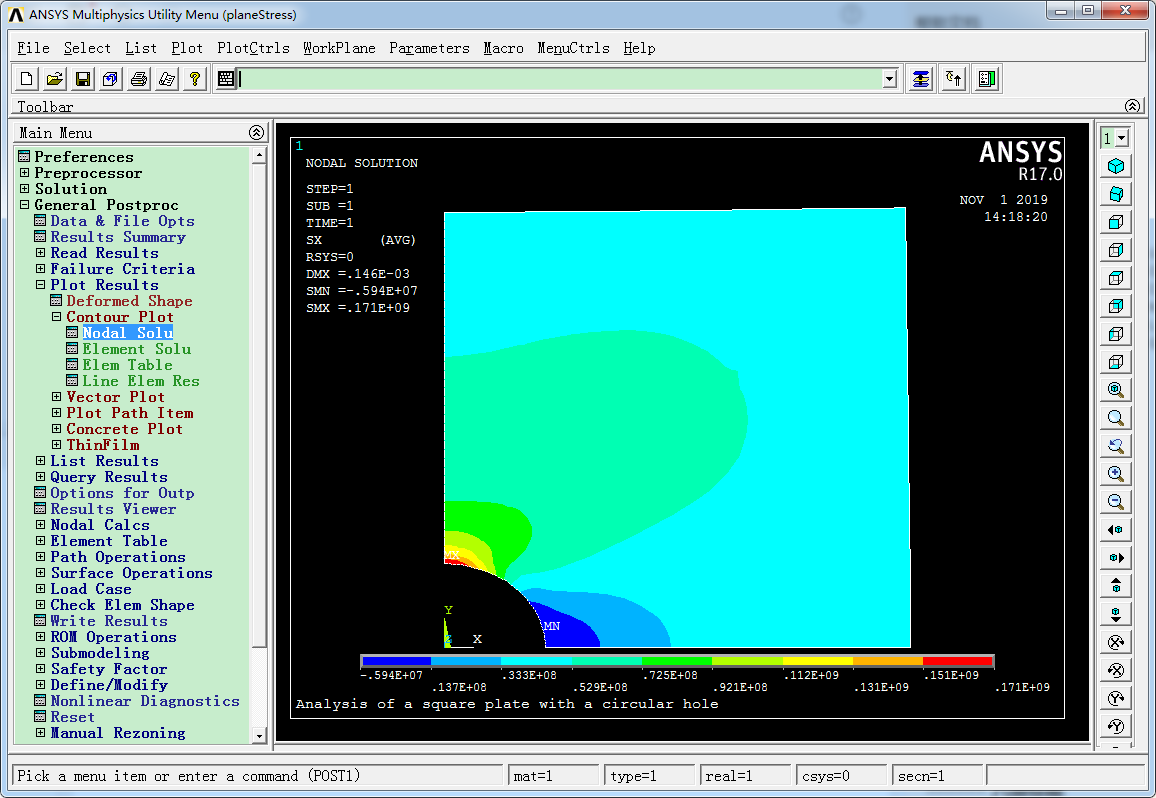可见，圆孔x方向的最大正应力位于最上部点，其大小为0.171e9Pa。
4.3 绘制路径上的x方向正应力曲线图
（a）定义路径
Main Menu中，Genreal Postproc -> Path Operations -> Define Path -> By Location，
定义名字为“midLine”，其余默认，OK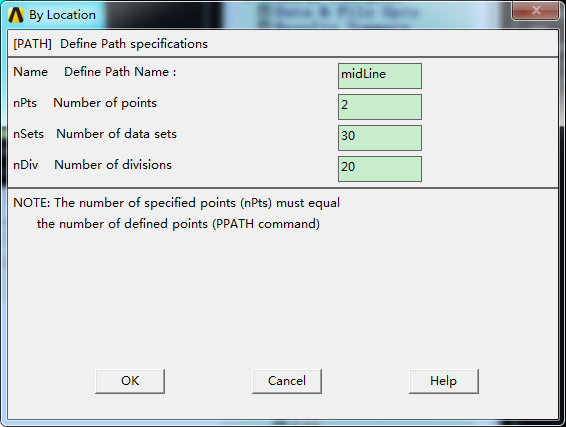输入编号为1的点，坐标为0,0.1,0，OK
输入编号为2的点，坐标为0,0.5,0，OK
然后Cancel，关闭对话框。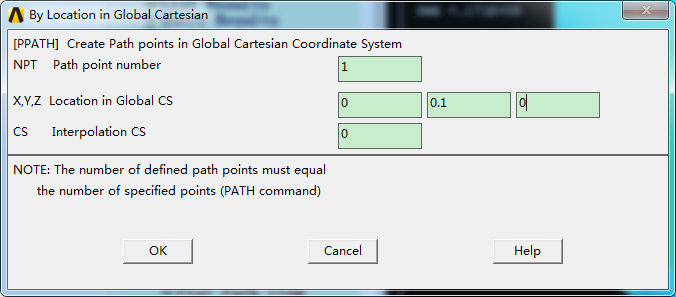（b）观察定义的路径
Main Menu中，Genreal Postproc -> Path Operations -> Define Path -> Plot Paths，
则图形中显示了刚才定义好的路径。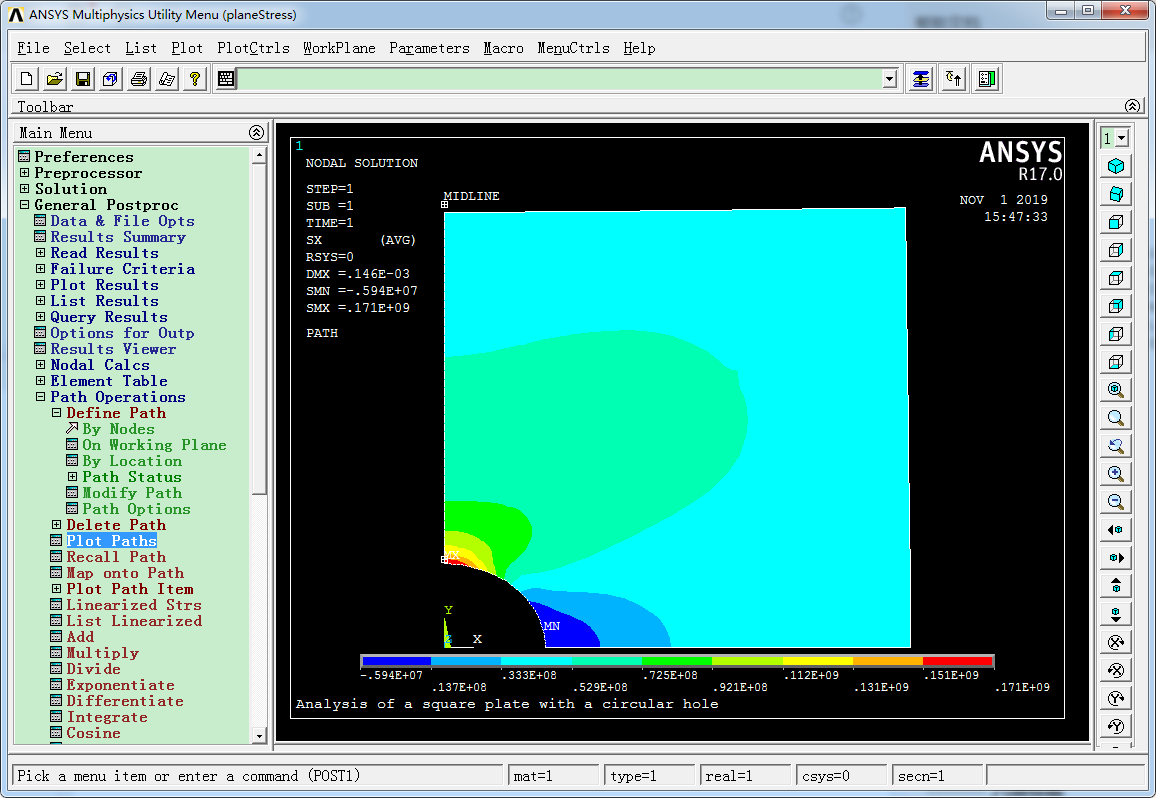（c）映射路径
Main Menu中，Genreal Postproc -> Path Operations -> Map onto Path，
Lab中输入名字“xstress”，双列表选择框中选择Stress和X-Direction，SX选项，OK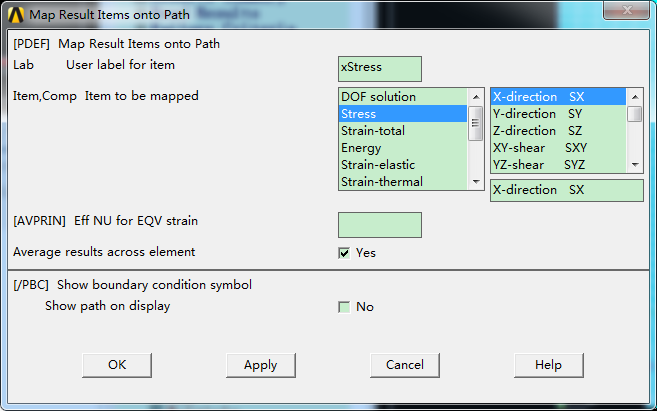（d）路径显示
显示x方向的正应力曲线
Main Menu中，Genreal Postproc -> Path Operations -> Plot Path Item -> On Graph，
选择XSTRESS，OK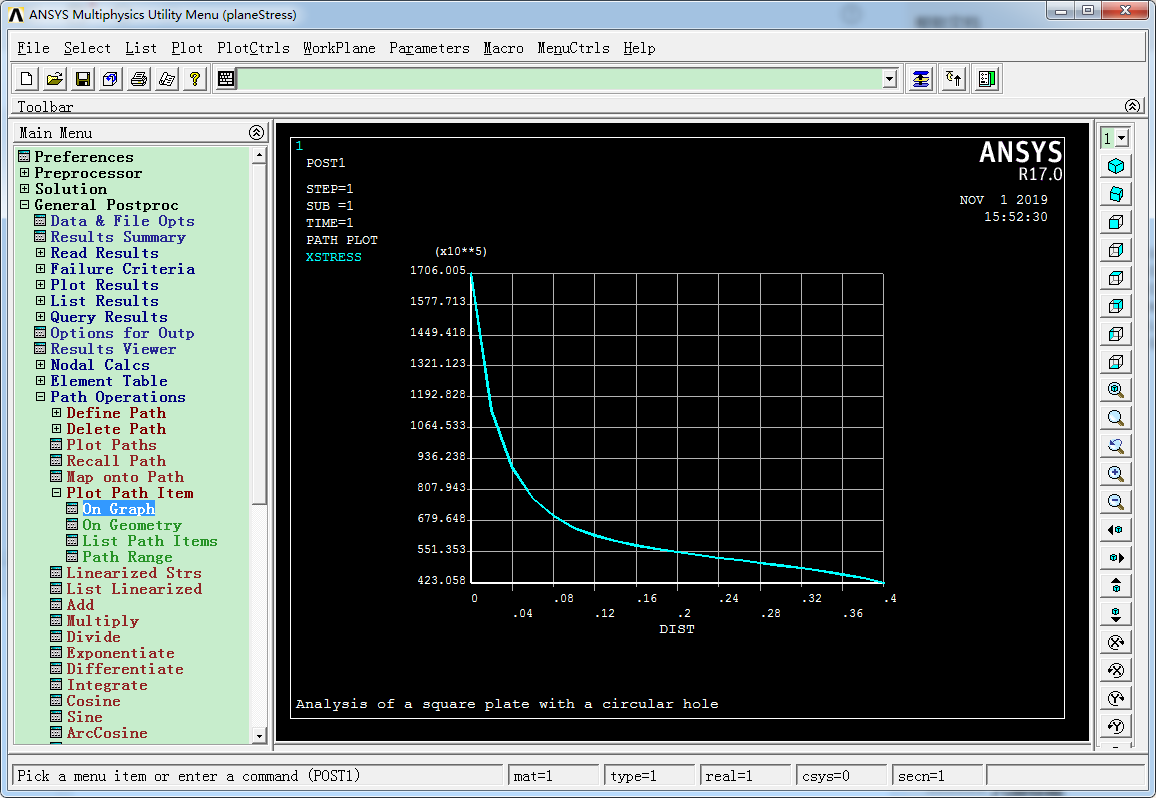则绘制出了中线上x方向的正应力曲线图。
那么孔处应力最大，为1706.005e5Pa，而远离孔处应力较小，壁板最上端的应力为423.058e5Pa。
实际上，这个问题并没有解析解，那么我们找到了一个近似问题的解析解，也就是含孔的无限大薄板，两侧受均布拉伸力q的情况下，是由解析形式的应力表达式的，根据这个表达式可以算出来，圆孔处x方向正应力为3q，而距离圆孔5倍半径处的x方向正应力约为1.0224q。
3q = 150MPa，1.0224q 约为 50MPa，ANSYS得出来的结果分别为171MPa和42MPa，大约和上述解在量级上吻合，考虑到精确解的问题只是本算例问题的近似，那么我们可以认为所得结果是可信的，当然，也可以进一步加密网格来验证网格无关性，得出更加准确的解。
5. 关闭软件


展开全文• 三维内应力分析目前常用冻结切片法和散光法进行测量。1970年Н.К.АЬеn提出改变入射光偏振状态或波长来求解各片层的平面应力.迄今,三维主应力σ_1,σ_2及σ_3的测量与分析还未见有完满的光学切片法。本文对板形受...
• 包含一个桁架机构算例和平面应力算例，均较为基础，代码后大多有注释，可供初学者参考
• 用谱随机有限元方法分析了弹性模量不确定并承受确定性分布载荷的二维平面应力实体。 此参考示例在 Ghanem 和 Spanos 的第 (5.3) 节：“随机有限元：谱方法”中进行了描述。 主函数“main_ssfem_plate.m”包含此问题...matlab
• 以二维模型为基础,对端面双向抽运双包层平面波导激光器的热效应进行了理论分析和模拟计算,并研究了影响双包层平面波导激光介质中热应力的因素。
• 简要分析了单块非平面环形腔单频激光器的基本原理,并采用琼斯矩阵计算了单块单向行波非平面环形腔内的本征偏振态。深入分析了磁场强度、输出面偏振反射系数、入射角、非平面角及腔内其它参量对各本征偏振态的损耗及...
• 根据Ｊ２流动理论并采用有限元法分析了小范围屈服条件下，各向同性幂强化材料及理想塑性材料中的准静态Ｉ型平面应力稳恒裂纹扩展问题。计算结果表明，与平面应变情况不同，对于平面应力稳恒裂纹扩展，材料的强化指数...
• 结果表明:平面应力状态下,由于构造应力的作用,最大主应力在与煤壁呈一定角度的位置应力集中明显,且沿煤壁水平方向出现一定程度的应力增大,而采空区方向应力卸压程度略微增大。因此,水平构造应力小于远场垂直应力的...
• 研究了正交异性双材料反平面平板搭接界面端...结果显示 :反平面搭接界面端只有一个奇异性,上下材料常数比Γ> 0时,应力场具有幂次奇异性,且随着Γ增长,奇异指数趋于 - 1/2,并利用有限元算例分析验证了理论结果的正确性。
• 该模型是描述等参三角形平面应力有限元模型。该单元具有恒定的厚度“th”，其他尺寸不需要。 假设每个角在“x”和“y”方向上分别具有两个自由度位移“u”和“v”。 因此，该单元具有节点力（Fx 和 Fy 的力对）和总...matlab
• 前面咱们讲得都是1维问题，下面咱们...在弹性力学中，常见的有3类2维问题，即，平面应力、平面应变、轴对称问题。咱们讲下什么是平面应力和平面应变问题。 关于平面应力和平面应变更加详细的弹性力学推导，请参考： ...
前面咱们讲的都是1维问题，下面咱们讲讲2维问题。实际上，所有的现实问题都是3维问题，可是在特定情况下，可以把它们简化为2维问题来分析。在弹性力学中，常见的有3类2维问题，即，平面应力、平面应变、轴对称问题。咱们讲下什么是平面应力和平面应变问题。
关于平面应力和平面应变更加详细的弹性力学推导，请参考：
https://wenku.baidu.com/view/c63e00c7aa00b52acfc7caf7.html
1 平面应力问题
对于一个薄板，即长度和宽度远远大于厚度的板，其边缘受平行于板面且不沿厚度方向变化的面力和体力，则可近似认为是平面应力问题。比如平板两端拉伸的问题，注意平板受弯曲的问题则不满足该条件，因其受力是垂直于板面的！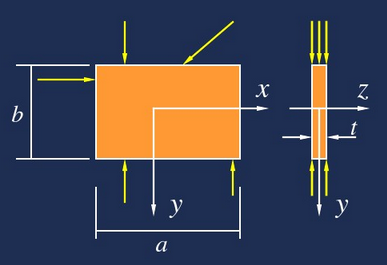原本的6个应力$\sigma_x$、$\sigma_y$、$\sigma_z$、$\tau_{xy}=\tau_{yx}$、$\tau_{yz}=\tau_{zy}$、$\tau_{zx}=\tau_{xz}$，
平面应力状态下，z方向应力近似为0，即，对于上面的薄板受力状态而言，其上下表面是没有z方向的应力的！即$\sigma_z=0$、$\tau_{zy}=0$、$\tau_{zx}=0$，
那么仅剩下$\sigma_x$、$\sigma_y$、$\tau_{xy}$三个应力，故而是平面应力状态！
2 平面应变问题
对于柱体，即长度远大于截面尺寸，柱面上承受平行于横截面且不沿长度方向变化的面力和体力，则可近似认为是平面应变问题。比如，水坝、厚壁圆筒、滚柱等问题。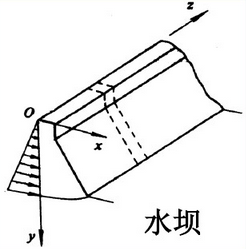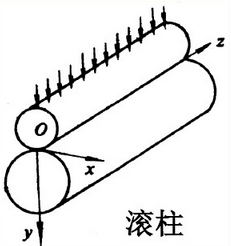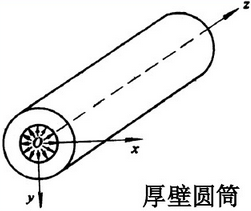由于z方向很长，其类似于周期边界，没有z方向的位移发生，所以z方向的应变$\epsilon_z=0$、$\gamma_{zx}=0$、$\gamma_{zy}=0$。
如此，原本三维空间的6个应变量$\epsilon_x$、$\epsilon_y$、$\epsilon_z$、$\gamma_{xy}=\gamma_{yx}$、$\gamma_{yz}=\gamma_{zy}$、$\gamma_{zx}=\gamma_{xz}$仅剩下了xy平面上的3个量，即$\epsilon_x$、$\epsilon_y$、$\gamma_{xy}$，所以叫做平面应变问题。
3 平衡方程（应力方程）
\left\{ \begin{aligned} \frac{\partial\sigma_x}{\partial x} + \frac{\partial\tau_{xy}}{\partial y}+X=0 \\ \frac{\partial\tau_{yx}}{\partial x} + \frac{\partial\sigma_y}{\partial y}+Y=0 \end{aligned} \right.
4 几何方程（应变位移方程）
\left\{ \begin{aligned} \epsilon_x=\frac{\partial u}{\partial x} \\ \epsilon_y=\frac{\partial v}{\partial y} \\ \gamma_{xy}=\frac{\partial u}{\partial y}+\frac{\partial u}{\partial x} \end{aligned} \right.
5 物理方程（应力应变关系）
（1）平面应力情况
$\sigma_x$、$\sigma_y$、$\tau_{xy}$ 与$\epsilon_x$、$\epsilon_y$、$\gamma_{xy}$、$\epsilon_z$的关系。
注意z方向没有应力，但是有个应变$\epsilon_z$！
\left\{ \begin{aligned} \epsilon_x=\frac{1}{E}(\sigma_x-\mu\sigma_y) \\ \epsilon_y=\frac{1}{E}(\sigma_y-\mu\sigma_x) \\ \gamma_{xy}=\frac{2(1+\mu)}{E}\tau_{xy} \end{aligned} \right.
$\epsilon_z$的求法
$\epsilon_z=\frac{\mu}{E}(\sigma_x+\sigma_y)$
（2）平面应变情况
$\sigma_x$、$\sigma_y$、$\sigma_z$、$\tau_{xy}$ 与$\epsilon_x$、$\epsilon_y$、$\gamma_{xy}$的关系。
注意z方向没有应变，但是有个应力$\sigma_z$！
\left\{ \begin{aligned} \epsilon_x=\frac{1-\mu^2}{E}(\sigma_x-\frac{\mu}{1-\mu}\sigma_y) \\ \epsilon_y=\frac{1-\mu^2}{E}(\sigma_y-\frac{\mu}{1-\mu}\sigma_x) \\ \gamma_{xy}=\frac{2(1+\mu)}{E}\tau_{xy} \end{aligned} \right.
$\sigma_z$的求法
$\sigma_z=\mu(\sigma_x+\sigma_y)$
不难发现，两者方程形式上是相同的，只是系数不同罢了！这也就是为什么它们放在一起讨论。
平衡方程2个 + 几何方程3个 + 物理方程3个 = 8个方程，未知量有8个，即$u$、$v$、$\epsilon_x$、$\epsilon_y$、$\gamma_{xy}$、$\sigma_x$、$\sigma_y$、$\tau_{xy}$，注意$\sigma_z$和$\epsilon_z$并不参加运算，其可由计算结果直接算出来。
那么方程组时封闭的，可解！
6 边界条件
受力边界条件
$\sigma_{ij}n_j=T_i$
位移边界条件
$u_i=\overline u_i$
7 有限元方法
单元及其形函数（三角单元、矩形单元），刚度矩阵推导等，较为复杂，不要求本科生掌握，故不再写出，感兴趣的可参考相关书籍资料。
咱们只要知道要分析的问题属于平面应力还是平面应变问题就好了，因为在ANSYS中，他们是用一个单元表示的，需要在单元属性中去指明是平面应力还是平面应变问题。


展开全文• 弹塑性力学1–应力分析(1) 弹性力学的研究对象和内容 ​ 物体受外载荷作用所产生的形状和大小的改变，称之为变形或形变，通常考虑的外部载荷包括机械外力、温度、电磁力等各种物理因素。如果将引起变形的外部载荷移...弹塑性力学 Cauchy应力公式 边界条件
• 应用有限元分析软件，对两种跨度下典型平面钢屋架节点刚性所产生的次应力进行了分析计算。对典型平面钢屋架采用了平面铰接模型、平面刚接模型I以及平面刚接模型Ⅱ。分析了在普通荷栽作用下钢屋架次应力的大小、分布...
• 本文介绍了某高砾石粘土心墙堆石坝平面有限元应力应变分析，对两种坝型采用不同本构模型进行了分析比较，特别对防渗心墙的拱效应、抗水力劈裂性能及砼防渗墙应力进行了深入的分析研究，并对水力劈裂的判断标准进行了...
• 应力分析（3）1 八面体上的剪应力与正应力 考虑物体中的一点，过该点作一外法线nnn与3个应力主方向有相同角度的斜面，它的3个方向余弦是 (l,m,n)=(±13,±13,±13) (l,m,n)=(\pm\frac{1}{\sqrt{3}},\pm\frac{1}{\...
• 以给定的混凝土强度准则为基础,给出了混凝土平面应力状态下的增量本构关系, 利用特征屈服半径的概念将初始屈服面、后继屈服面以及破坏面表达为一个统一的形式;特征屈服半径的概念将平面应力状态下混凝土的本构关系与...
• 128×128元锑化铟红外焦平面探测器热-应力耦合分析研究论文
• 方法基于微观层次的混凝土本构模型———微平面模型原理，将其与具有优越非线性分析能力的通用有限元程序MSC.MARC相结合，开发了相关程序，并对混凝土在单轴、双轴、三轴、往复受力等各种应力状态下的行为和一片实际......